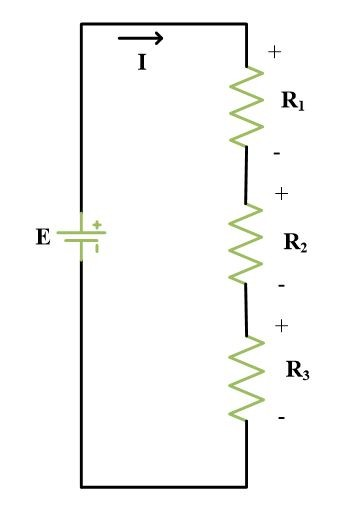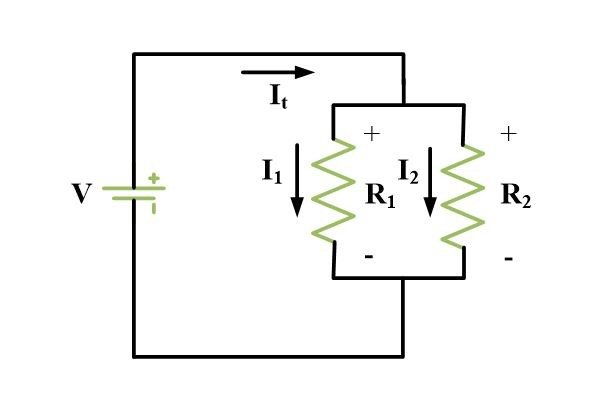Home / Basic Electrical / Voltage divider Circuits and Current divider Circuits

# Voltage divider Circuits and Current divider Circuits

Want create site? Find Free WordPress Themes and plugins.

In analyzing a series circuit, it becomes necessary to find voltage drop across one or more of the resistances. A simple voltage drop relationship may be obtained by referring to the following figure.The total current is given by,

$I=\frac{E}{{{R}_{1}}+{{R}_{2}}+{{R}_{3}}}$

And the voltage drop are given by,

${{V}_{1}}=I{{R}_{1}}=E\frac{{{R}_{1}}}{{{R}_{1}}+{{R}_{2}}+{{R}_{3}}}~~~~\text{ }~~~\left( 1 \right)$

${{V}_{2}}=I{{R}_{2}}=E\frac{{{R}_{2}}}{{{R}_{1}}+{{R}_{2}}+{{R}_{3}}}~~\text{ }~~~~~~\left( 2 \right)$

${{V}_{3}}=I{{R}_{3}}=E\frac{{{R}_{3}}}{{{R}_{1}}+{{R}_{2}}+{{R}_{3}}}~~\text{ }~~~~~~\left( 3 \right)$

Notice in these relationships that a voltage drop across a resistor is proportional to the ratio of that resistance to the total resistance of that circuit. A general statement called the voltage divider rule can be made as follows:

In a series circuit, the voltage drop across a particular resistor Rn is the source voltage times the fraction Rn/Rt.

${{V}_{n}}=E\frac{{{R}_{n}}}{{{R}_{t}}}$

In the above expression, E expresses source voltage and Ris the total circuit resistance.

## Example of Voltage Division

Determine the voltage drops V1, V2, and V3 in the circuit of the following Fig.Solution

The total resistance

${{R}_{t}}=60+85+55=200~\Omega$

Then, by equations 1, 2 and 3

${{V}_{1}}=E\frac{{{R}_{1}}}{{{R}_{1}}+{{R}_{2}}+{{R}_{3}}}=10*\frac{60}{200}=3~V$

${{V}_{2}}=E\frac{{{R}_{2}}}{{{R}_{1}}+{{R}_{2}}+{{R}_{3}}}=10*\frac{85}{200}=4.25~V$

${{V}_{3}}=E\frac{{{R}_{3}}}{{{R}_{1}}+{{R}_{2}}+{{R}_{3}}}=10*\frac{55}{200}=2.75~V$

A check by Kirchhoff’s voltage law indicates that

${{V}_{1}}+{{V}_{2}}+{{V}_{3}}=10~V=E$

## Current Divider Circuit

Resistors in parallel lend itself to a current division rule. In the following figure, the total current coming to the parallel combination of R1 and R2 divides into the currents I1 and Irespectively.The branch currents are:

${{I}_{1}}=\frac{V}{{{R}_{1}}}$

${{I}_{2}}=\frac{V}{{{R}_{2}}}$

However, the voltage drop V equals ItRt where, Rt is given by the following equation

${{R}_{t}}=\frac{{{R}_{1}}{{R}_{2}}}{{{R}_{1}}+{{R}_{2}}}$

So, the voltage drop is,

$V={{I}_{t}}{{R}_{t}}={{I}_{t}}\frac{{{R}_{1}}{{R}_{2}}}{{{R}_{1}}+{{R}_{2}}}$

Substitute V into branch currents, we have

${{I}_{1}}={{I}_{t}}\frac{{{R}_{2}}}{{{R}_{1}}+{{R}_{2}}}~~~~~~~\text{ }\cdots \text{ }~~~~\left( 4 \right)$

${{I}_{2}}={{I}_{t}}\frac{{{R}_{1}}}{{{R}_{1}}+{{R}_{2}}}~~~~~~~~\text{ }\cdots \text{ }~~~~\left( 5 \right)$

Equations (4) and (5) are mathematical statements for current divider rule, which is stated as follows:

“With two resistors in parallel, the current in either resistor is the total current times the ratio of the opposite resistor over the sum of the two resistors.”

## Example of current division

Find the branch currents I1 and I2  for the parallel circuit of the following Fig.Solution

By the current division principle,

${{I}_{1}}={{I}_{t}}\frac{{{R}_{2}}}{{{R}_{1}}+{{R}_{2}}}=20mA*\frac{5K}{5K+10K}=6.7~mA$

${{I}_{2}}={{I}_{t}}\frac{{{R}_{1}}}{{{R}_{1}}+{{R}_{2}}}=20mA*\frac{10K}{5K+10K}=13.3~mA$

In above example, notice that since R1 is twice the resistance of R2, one-half as much current will flow in it as in R2. Thus is any parallel circuit, the ratio between any two branch currents equals the inverse of their resistance ratio.

Did you find apk for android? You can find new Free Android Games and apps.